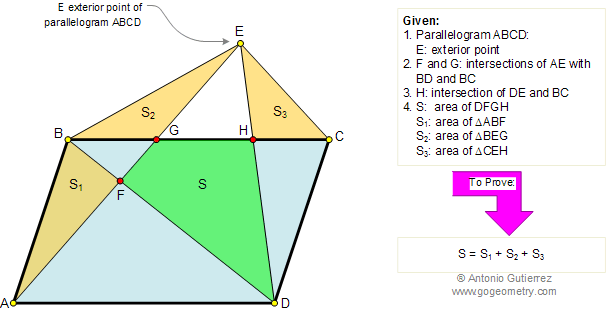# Geometry Problem 167. Parallelogram, Diagonal, Triangles, Areas. Level: High School, Honors Geometry, College, Mathematics Education

In the figure below, ABCD is a parallelogram with E an exterior point. F and G are the points of intersection of AE with BD and BC. H is the point of intersection of DE and BC. If S, S1, S2, and S3 are the areas of quadrilateral DFGH, and triangles ABF, BEG, and CEH respectively, prove that S = S1 + S2 + S3 .
View or post a solutionHome | Geometry | Search | Problems | 161-170 | Area of a Parallelogram | Email | By Antonio Gutierrez